# 06-1350/Class Notes for Tuesday November 7

## The Fundamental Theorem of Finite Type Invariants

Almost Theorem. There exists a universal TG-morphism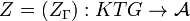$Z=(Z_\Gamma):KTG\to{\mathcal A}$ from the TG-algebra of knotted trivalent graphs to the TG-algebra of Jacobi diagrams. Furthermore, any two such TG-morphisms are twist equivalent.

Theorem. (Essentially due to Murakami and Ohtsuki, [MO]; see also Dancso [Da]) There exists an R-normal TG-morphism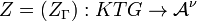$Z=(Z_\Gamma):KTG\to{\mathcal A}^\nu$ from the TG-algebra of knotted trivalent graphs to the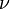$\nu$-twisted TG-algebra of Jacobi diagrams. Furthermore, any two such TG-morphisms are twist equivalent.

The above theorem is simply the accurate formulation of the almost theorem above it. The "almost theorem" is just what you would have expected, with an additional uniqueness statement. The "theorem" just adds to it a few normalizations that actually make it right. The determination of these normalizations is quite a feat; even defining them takes a page or two. I'm not entirely sure why the Gods of mathematics couldn't have just allowed the "almost theorem" to be true and make our lives a bit simpler.

Enough whining; we just need to define "R-normal" and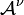${\mathcal A}^\nu$.

Definition.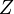$Z$ is called R-normal if Failed to parse (unknown function\MobiusSymbol): Z(\bigcirc)^{-1}Z(\MobiusSymbol)=\exp(\isolatedchord/4)

in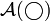${\mathcal A}(\bigcirc)$, where Failed to parse (unknown function\MobiusSymbol): (\MobiusSymbol)
denotes the positively-twisted Möbius band and where Failed to parse (unknown function\isolatedchord): (\isolatedchord)
denotes the unique degree 1 chord diagram in${\mathcal A}(\bigcirc)$.


Definition.${\mathcal A}^\nu$ is almost the same as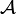${\mathcal A}$. It has the same spaces (i.e., for any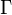$\Gamma$,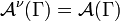${\mathcal A}^\nu(\Gamma)={\mathcal A}(\Gamma)$), but the unzip operations on${\mathcal A}^\nu$ get "renormalized":

The edge-unzip operations.
Let$\nu$ denote the specific element of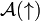${\mathcal A}(\uparrow)$ defined in the following subsection. If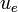$u_e$ denotes the unzip operation of an edge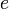$e$ for the TG-algebra${\mathcal A}$ and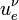$u^\nu_e$ is the corresponding operation in${\mathcal A}^\nu$, the two operations are related by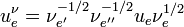$u^\nu_e=\nu^{-1/2}_{e'}\nu^{-1/2}_{e''}u_e\nu^{1/2}_e$. Here "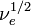$\nu^{1/2}_e$" means "inject a copy of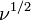$\nu^{1/2}$ on the edge$e$ of$\Gamma$, and likewise, "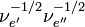$\nu^{-1/2}_{e'}\nu^{-1/2}_{e''}$" means "inject copies of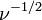$\nu^{-1/2}$ on the edges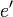$e'$ and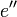$e''$ of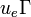$u_e\Gamma$ that are created by the unzip of$e$".

### The Mysterious$\nu$

It remains to define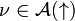$\nu\in{\mathcal A}(\uparrow)$. Well, it is the element often called "the invariant of the unknot", for indeed, by a long chain of reasoning, it is the invariant of the unknot. It is also given by the following explicit formula of [BGRT] and [BLT]: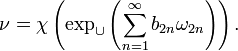$\nu=\chi\left(\exp_\cup\left(\sum_{n=1}^\infty b_{2n}\omega_{2n}\right)\right).$

In the above formula \chi denotes the PBW "symmetrization" map,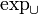$\exp_\cup$ means "exponentiation in the disjoint union sense",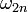$\omega_{2n}$ is the "wheel with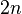$2n$ legs" (so Failed to parse (unknown function\twowheel): \omega_2=\twowheel,

Failed to parse (unknown function\fourwheel): \omega_4=\fourwheel,
etc.) and the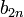$b_{2n}$'s are the "modified Bernoulli numbers" defined by the power series expansion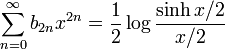$\sum_{n=0}^\infty b_{2n}x^{2n} = \frac12\log\frac{\sinh x/2}{x/2}$

(so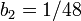$b_2=1/48$,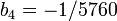$b_4=-1/5760$,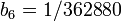$b_6=1/362880$, etc.).

### Some values of the invariant

While these are not necessary for the statement of the theorem, it is worthwhile to note that the invariants of the unknot, the unknotted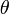$\theta$-graph and the unknotted dumbbell are as follows: